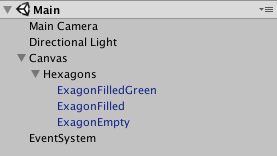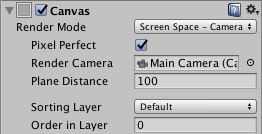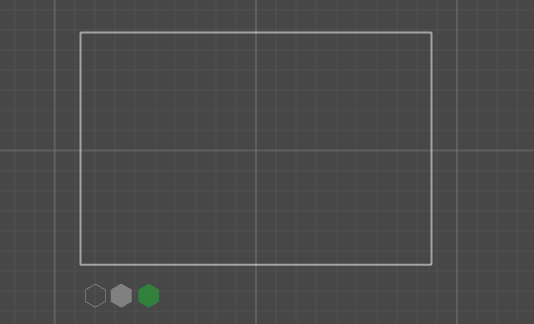# Unity3D – Draw hexagons map in canvas

Today, a code extract from one of my 3d games… (Viruz!, download here), an hexagons map.

### What we need before?

1. Unity 3D
2. Some hexagons prefabs
1. draw it by yourself
2. draw at runtime using code (example here)

### Final result:You need to add on your canvas the hexagons prefab first (i’ve used also an empty group called “Hexagons”):Next setup your canvas to use the “Screen Space – Camera” and add on it the Main Camera if you want to use this script with a multi-size camera and different devices (both desktop and mobile app).Move your hexagons out of screen (we need it only to clone, so move outside).### Ok, give me the code!

Sorry, here the sample code…

[code lang=”csharp” autolinks=”false” collapse=”false” firstline=”1″ gutter=”true” htmlscript=”false” light=”false” padlinenumbers=”false” smarttabs=”true” tabsize=”4″ toolbar=”false”]
using&nbsp; UnityEngine;
using&nbsp; System.Collections;
using&nbsp; UnityEngine.UI;
public&nbsp; class&nbsp; ExagonGenerator&nbsp;: &nbsp;MonoBehaviour {
// total hex drawed
int&nbsp; drawedHexagons&nbsp; = &nbsp;0;

// the 3 colored random hexagons
int&nbsp; randomHexPos_1&nbsp; = &nbsp;0;
int&nbsp; randomHexPos_2&nbsp; = &nbsp;0;
int&nbsp; randomHexPos_3&nbsp; = &nbsp;0;

int&nbsp; randomHexPos_Max&nbsp; = &nbsp;100;

// the hex prefabs
GameObject exagonEmpty;
GameObject exagonFilled;
GameObject exagonGreen;

void&nbsp; Start() {
// called from here (or initialized using a public var from editor)
exagonEmpty&nbsp; = &nbsp;GameObject.Find("ExagonEmpty");
exagonFilled&nbsp; = &nbsp;GameObject.Find("ExagonFilled");
exagonGreen&nbsp; = &nbsp;GameObject.Find("ExagonFilledGreen");

// random positions
randomHexPos_1&nbsp; = &nbsp;Random.Range(5, randomHexPos_Max);
randomHexPos_2&nbsp; = &nbsp;Random.Range(5, randomHexPos_Max);
randomHexPos_3&nbsp; = &nbsp;Random.Range(5, randomHexPos_Max);

Draw();
}

void&nbsp; Draw() {
// size
RectTransform exagonRect&nbsp; = &nbsp;exagonGreen.GetComponent();
RectTransform canvasRect&nbsp; = &nbsp;gameObject.GetComponent();

// space between hex
float&nbsp; delta&nbsp; = &nbsp;exagonRect.rect.width&nbsp; + &nbsp;10;

int&nbsp; counter&nbsp; = &nbsp;0;
// looping the matrix
for&nbsp; (float&nbsp; y&nbsp; = &nbsp;canvasRect.rect.y;&nbsp; y&nbsp; &amp; lt;&nbsp; Mathf.Abs(canvasRect.rect.y)&nbsp; + &nbsp;delta;&nbsp; y&nbsp; += &nbsp;delta) {
for&nbsp; (float&nbsp; x&nbsp; = &nbsp;canvasRect.rect.x;&nbsp; x&nbsp; &amp; lt;&nbsp; Mathf.Abs(canvasRect.rect.x);&nbsp; x&nbsp; += &nbsp;delta) {
float&nbsp; fixedX&nbsp; = &nbsp;x;
if&nbsp; (counter&nbsp; % &nbsp;2&nbsp; == &nbsp;0)&nbsp; // make it disaligned
{
fixedX&nbsp; += &nbsp;(delta&nbsp; / &nbsp;2);
}

// the hex to draw
GameObject exagon&nbsp; = &nbsp;Random.Range(1, &nbsp;4)&nbsp; % &nbsp;2&nbsp; == &nbsp;0&nbsp; ? &nbsp;exagonFilled&nbsp; : &nbsp;exagonEmpty;
if&nbsp; (drawedHexagons&nbsp; == &nbsp;randomHexPos_1&nbsp; || &nbsp;drawedHexagons&nbsp; == &nbsp;randomHexPos_2&nbsp; || &nbsp;drawedHexagons&nbsp; == &nbsp;randomHexPos_3) {
exagon&nbsp; = &nbsp;exagonGreen;
}
drawedHexagons++;

Vector3 pos&nbsp; = &nbsp;new&nbsp; Vector3(fixedX, y, &nbsp;0);